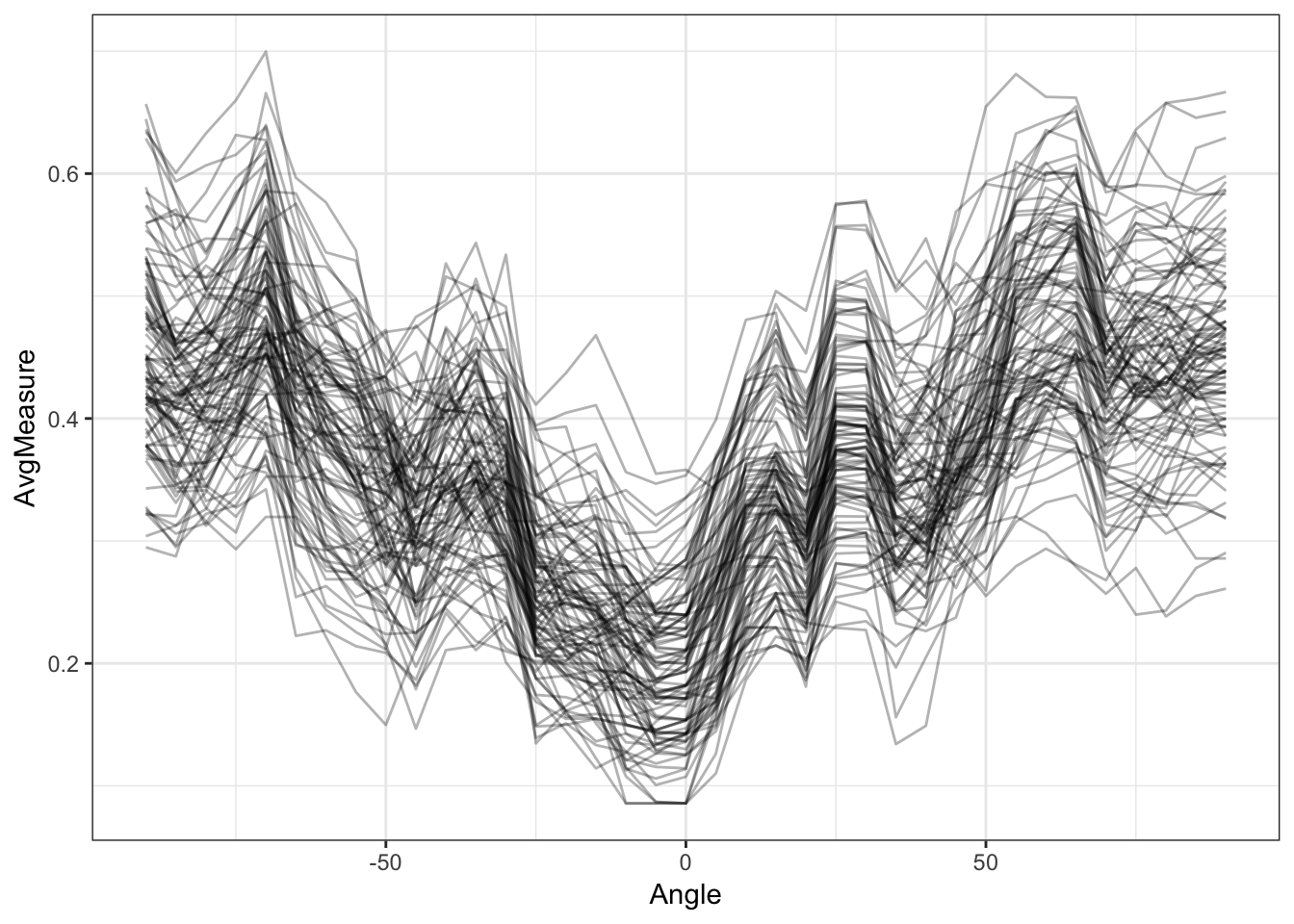# Bootstrapping clustered data

When evaluating the sampling variability of different statistics, I’ll often use the bootstrap procedure to resample my data, compute the statistic on each sample, and look at the distribution of the statistic over several bootstrap samples.

In principle, the bootstrap is straightforward to do. However, if you have correlated data (like repeated measures or longitudinal data or circular data), the unit of sampling no longer is the particular data point but the second-level unit within which the data are correlated; otherwise you break the correlation structure of the data by doing a naive bootstrap and distort the resultant distributions. This procedure is often called the cluster bootstrap.

Let’s fix ideas using a data analysis I’m currently doing. We’re looking at a particular measurement taken around a spinal joint every 5 degrees. These measures are correlated within person, since the measurements share the common spine. So to bootstrap our dataset, we have to bootstrap the people and not the individual measurements. A few rows of the data are below:

ID Angle Measure
16 -90 1
16 -85 1
16 -80 1
16 -75 1
16 -70 1
16 -65 1

The Measure variable varies from 0 to 1. The Angle variable varies between -90 and 90 by 5 degree increments.

Doing this computation is not difficult, but it becomes really straightforward using the rsample package developed by the RStudio crew, specifically Max Kuhn and Hadley Wickham. I was recently in a workshop Max taught in DC, where he introduced me to the rsample package, which, conveniently, has a bootstraps function. Now, this function has an option strata that can do stratified sampling. However, that is not the right tool, since that would sample from the individual measurements, just separately sampling by stratum. What we do need is to sample by the individuals.

Note that the bootstraps function samples rows from a data.frame or tibble object. In our situation, we need to sample groups of rows corresponding to each unique ID. However, we can utilize list-columns in tibbles to transform groups of rows into, effectively, single rows.

D <- d %>% nest(-ID)
head(D)
## # A tibble: 6 x 2
##      ID data
##   <dbl> <list>
## 1    16 <tibble [37 × 2]>
## 2    22 <tibble [37 × 2]>
## 3    38 <tibble [37 × 2]>
## 4    44 <tibble [37 × 2]>
## 5    30 <tibble [37 × 2]>
## 6    41 <tibble [37 × 2]>

Now, we can use bootstraps on this new, compact tibble to sample by ID

library(rsample)
set.seed(154234)

bs <- bootstraps(D, times = 10)
bs
## # Bootstrap sampling
## # A tibble: 10 x 2
##    splits          id
##    <list>          <chr>
##  1 <split [35/13]> Bootstrap01
##  2 <split [35/13]> Bootstrap02
##  3 <split [35/14]> Bootstrap03
##  4 <split [35/12]> Bootstrap04
##  5 <split [35/13]> Bootstrap05
##  6 <split [35/11]> Bootstrap06
##  7 <split [35/12]> Bootstrap07
##  8 <split [35/11]> Bootstrap08
##  9 <split [35/12]> Bootstrap09
## 10 <split [35/14]> Bootstrap10

You can read up about the rsplit object and how data is stored in this object here. Let’s look at one of these bootstrap samples:

as.tibble(bs$splits[]) %>% arrange(ID) %>% head() ## Warning: as.tibble() is deprecated, use as_tibble() (but mind the new semantics). ## This warning is displayed once per session. ## # A tibble: 6 x 2 ## ID data ## <dbl> <list> ## 1 2 <tibble [37 × 2]> ## 2 7 <tibble [37 × 2]> ## 3 8 <tibble [37 × 2]> ## 4 9 <tibble [37 × 2]> ## 5 9 <tibble [37 × 2]> ## 6 9 <tibble [37 × 2]> Notice that some ID’s are sampled multiple times, while others, not at all, which is the nature of bootstrap sampling. If we want to assess the bootstrap distribution of the average Measure for each Angle, we can just unnest this tibble, and then assess the averages by Angle. This would give one bootstrap sample. as.tibble(bs$splits[]) %>% unnest() %>%
group_by(Angle) %>% summarize(AvgMeasure = mean(Measure))
## # A tibble: 37 x 2
##    Angle AvgMeasure
##    <dbl>      <dbl>
##  1   -90      0.596
##  2   -85      0.557
##  3   -80      0.539
##  4   -75      0.532
##  5   -70      0.595
##  6   -65      0.530
##  7   -60      0.495
##  8   -55      0.480
##  9   -50      0.439
## 10   -45      0.383
## # … with 27 more rows

We can now use purrr functions to get the bootstrap distribution over multiple bootstrap samples, and plot the sampled summaries against Angle.

library(purrr)
library(ggplot2)
bs <- bootstraps(D, times = 100)
bs_AvgMeasure <- map(bs\$splits, ~as.tibble(.) %>% unnest %>% group_by(Angle) %>%
summarize(AvgMeasure = mean(Measure))) %>%
bind_rows(.id = 'boots')
ggplot(bs_AvgMeasure, aes(Angle, AvgMeasure, group = boots))+
geom_line(alpha= 0.3)+
theme_bw()